## A Rainy Day in TIC-80 City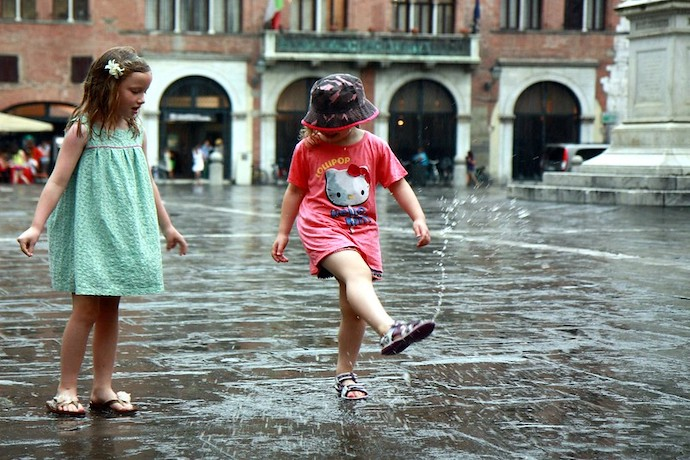Welcome back for another art adventure in TIC-80, the fantasy computer that provides an all-in-one system for making games, animations, and music. Last time, with this code, we made a field of stars that slowly scrolled down the screen.

``````stars = {}

function makeNewStar(top,bottom)
local newStar = {}
newStar.x = math.random(0,239)
newStar.y = math.random(top,bottom)
newStar.r = math.random(0,1)
newStar.t = math.random(0,240)
newStar.vy = math.random()/2 + 0.1
table.insert(stars,newStar)
end

for i=1,500 do
makeNewStar(0,136)
end

function TIC()
cls(0)

for i,s in ipairs(stars) do
circ(s.x,s.y,s.r,12)
s.y = s.y + s.vy
if s.t == 0 then
s.r = (s.r + 1)%2
s.t = 240
else
s.t = s.t-1
end

if s.y > 136 then
table.remove(stars,i)
makeNewStar(-20,-5)
end
end

end``````

Now we’re going to build on this code but change the setting to a little city scene with rainfall.

As always, start by making a new program with new lua at the TIC-80 command line and hitting ESC to enter the editor. I’ll go quickly over the parts that are like our last project then get to the fun differences that let us make something new.

First, start by clearing out all the existing code and making it look like the following, which is going to set up our code so that there’s rain falling down on the screen.

``````raindrops = {}

function makeDrop(x,y)
vy = math.random()*0.5+0.4
table.insert(raindrops,{x=x,y=y,vy=vy})
end

for i=1,200 do
makeDrop(math.random(-40,280),
math.random(-100,100))
end

function TIC()
cls(8)

for i,r in ipairs(raindrops) do
r.y = r.y + r.vy

line(r.x,r.y,r.x,r.y+3,12)

if r.y > 135
then
table.remove(raindrops,i)
makeDrop(math.random(-40,280),
math.random(-10,-5))
end
end
end``````

The only real difference between this and the starfield is that we’re drawing a line not a circle, but otherwise the rest is basically the same.

Now, we’re going to lay down a sidewalk. We’ll do this in a slightly modular way where we can easily play with the height and width of the rectangular segments of the sidewalk:

``````raindrops = {}

sidewalkHeight = 105

numSegments = 6

function makeDrop(x,y)
vy = math.random()*0.5+0.4
table.insert(raindrops,{x=x,y=y,vy=vy})
end

for i=1,200 do
makeDrop(math.random(-40,280),
math.random(-100,100))
end

function TIC()
cls(8)

--drawing the sidewalk
for i=1,numSegments do
local xSeg = 240 / numSegments
rect((i-1)*xSeg,sidewalkHeight,
xSeg,136-sidewalkHeight,14)
rectb((i-1)*xSeg,sidewalkHeight,
xSeg,136-sidewalkHeight,0)
end

for i,r in ipairs(raindrops) do
r.y = r.y + r.vy

line(r.x,r.y,r.x,r.y+3,12)

if r.y > 135
then
table.remove(raindrops,i)
makeDrop(math.random(-40,280),
math.random(-10,-5))
end
end
end``````

We put in the sidewalk drawing code before we draw the raindrops so that we see the rain is on top of the sidewalk and not covered up by it.

The next little feature we’re going to introduce is changing the line if r.y > 135 to if r.y > sidewalkHeight and math.random( ) < 0.15 so the rain starts disappearing randomly when it’s overlapped with the sidewalk. This gives the illusion that it’s falling onto different parts of the sidewalk, an easy way to convey depth. If we wanted to we could make this more sophisticated by making the “background” rain (the rain that’s falling slower) land further “back” on the sidewalk and let the “foreground” rain fall closer to the bottom of the screen.

Next, we’ll add puddles. The puddles will reflect the sky in a fun effect.

First, we’ll add the code to add puddles to another list, then we’ll add the code that makes some puddles on the sidewalk. Finally, we’ll do the clever part where we have the puddles reflect the sky. For space, I’ll cut the code we’ve seen before and put a comment that says –cut instead.

The clever trick here is that we split the drawing of the puddles into two pieces: a part before we draw the rain and a part after we draw the rain.

For the part before the rain, we draw circles with the data in the puddles list and draw them, for convenience, the same color as the sky. In the part after the rain, we use some tricks involving the pix function, which in TIC-80 lets you get the color of a pixel at a point if you give it two arguments, and set the color of a pixel at a point if you give it three arguments.

The logic is that we look for any points that are the color of the sky in the sidewalk, since those have to be puddles, and then we fill in those pixels with the color of the pixel that’s mirrored above the sidewalk.

``````raindrops = {}

puddles = {}

numSegments = 6

sidewalkHeight = 105

function makePuddle(x,y,r)
table.insert(puddles,{x=x,y=y,r=r})
end

makePuddle(30,110,15)
makePuddle(40,120,10)

makePuddle(179,118,5)
makePuddle(182,117,5)
makePuddle(181,114,4)

makePuddle(150,110,6)
--cut

function TIC()
-- cut

for _,p in ipairs(puddles) do
circ(p.x,p.y,p.r,8)
end

-- cut

for i=0,239 do
for j=sidewalkHeight,136 do
if pix(i,j) == 8 then
local yAbove = 2*sidewalkHeight - j
local cAbove = pix(i,yAbove)
pix(i,j,cAbove)
end
end
end

end``````

The last part is adding wind. It’s pretty straightforward, but it does mean we need a bit of trigonometry. The line of the rain must point in the direction the rain is moving, so we first need to calculate how much of the falling is to the side and how much is down. From there, we can figure out the slope of the line. Our final code looks like

``````t=0

raindrops = {}

puddles = {}

windV = 0.2

numSegments = 6

sidewalkHeight = 105

function makePuddle(x,y,r)
table.insert(puddles,{x=x,y=y,r=r})
end

makePuddle(30,110,15)
makePuddle(40,120,10)

makePuddle(179,118,5)
makePuddle(182,117,5)
makePuddle(181,114,4)

makePuddle(150,110,6)

function makeDrop(x,y)
vy = math.random()*0.5+0.4
table.insert(raindrops,{x=x,y=y,vy=vy})
end

for i=1,200 do
makeDrop(math.random(-40,280),
math.random(-100,100))
end

function TIC()

windV = 0.3*math.sin(t/500)

cls(8)

-- this is where we draw the sidewalk
for i=1,numSegments do
local xSeg = 240 / numSegments
rect((i-1)*xSeg,sidewalkHeight,
xSeg,136-sidewalkHeight,14)
rectb((i-1)*xSeg,sidewalkHeight,
xSeg,136-sidewalkHeight,0)
end

for _,p in ipairs(puddles) do
circ(p.x,p.y,p.r,8)
end

for I,r in ipairs(raindrops) do
r.y = r.y + r.vy
r.x = r.x + windV

local totalV = math.sqrt(windV^2 + r.vy^2)
line(r.x,r.y,r.x+3*windV/totalV,r.y+3*r.vy/totalV,12)

if r.y > sidewalkHeight and math.random() < 0.15
then
table.remove(raindrops,i)
makeDrop(math.random(-40,280),
math.random(-10,-5))
end
end

for i=0,239 do
for j=sidewalkHeight,136 do
if pix(i,j) == 8 then
local yAbove = 2*sidewalkHeight - j
local cAbove = pix(i,yAbove)
pix(i,j,cAbove)
end
end
end

t=t+1
end``````

Next time, we’ll get into some visual effects and music composition to make your programs full A/V art pieces.

#### Learning TIC80

https://tic80.com/learn

#### TIC80

https://tic80.com/

#### Learning to Program in TIC80

https://www.carnaghan.com/learning-to-program-in-tic-80-a-fantasy-console/

#### TIC80 Fantasy Computer

https://nesbox.itch.io/tic80

## Author

•Clarissa has worked in mathematics, physics, and computer science research but spends much of her time now trying to make computer science education accessible to a broader audience.

### Also In The February 2023 IssueIf aliens exist, why haven’t we met any yet?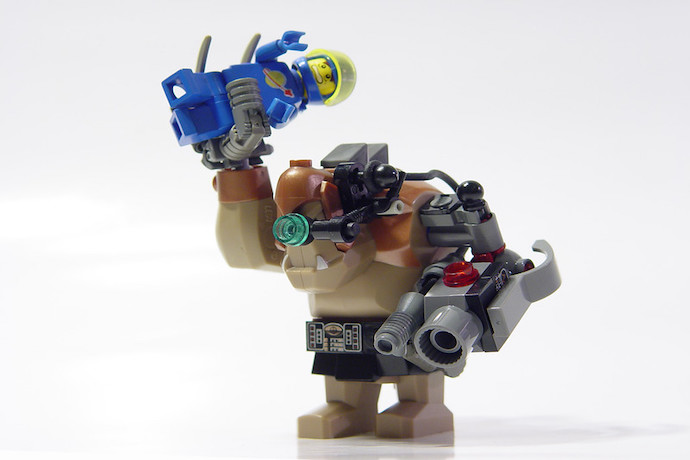Learn how the origins of cybernetics may pave the way for the future of machine learningDon’t let the name fool you, this systems programming language is anything but rusty!With every end, there is a new beginning. Especially in creative robotics!Don’t forget your umbrella for this TIC-80 Lab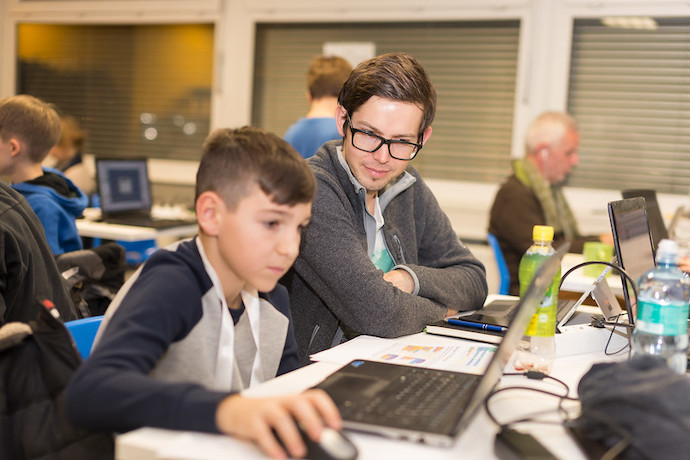Save time with this neat OpenSCAD shortcutTime to get inspired by mother nature!Learn how drones are being used to repair the Great Wall of China and find ancient structures!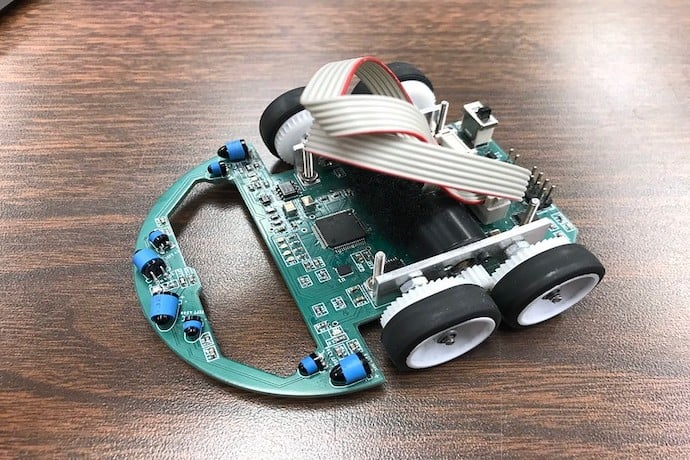These robot mice move like mechanical cats are chasing them!Try out this Zombie Survival mod for Minecraft if you dare!This trash loving AI joins the fight for a greener earth!Spice up your 3D-printed food with time!Now that you have made your masterpiece, it is time to share it with the world.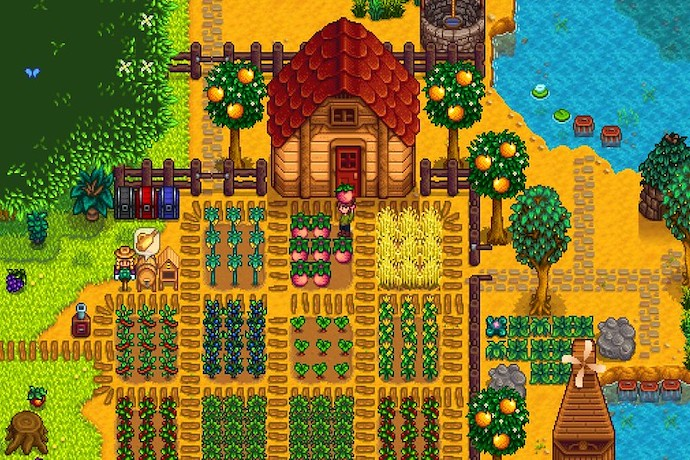Time to get your Farming robots’ hands dirty!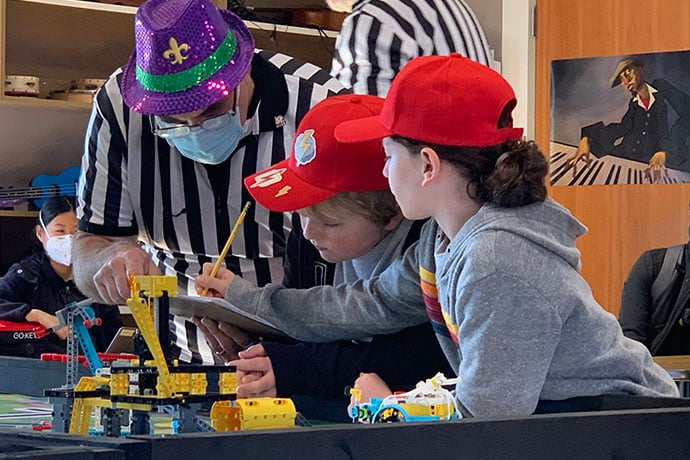Be the FIRST at your school to join this awesome program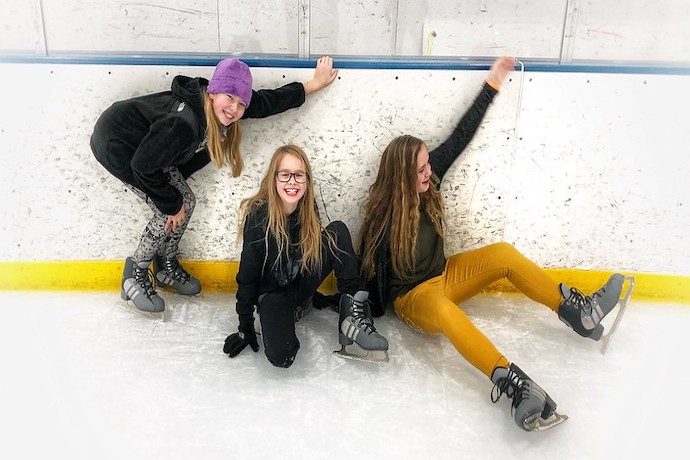Links from the bottom of all the February 2023 articles, collected in one place for you to print, share, or bookmark.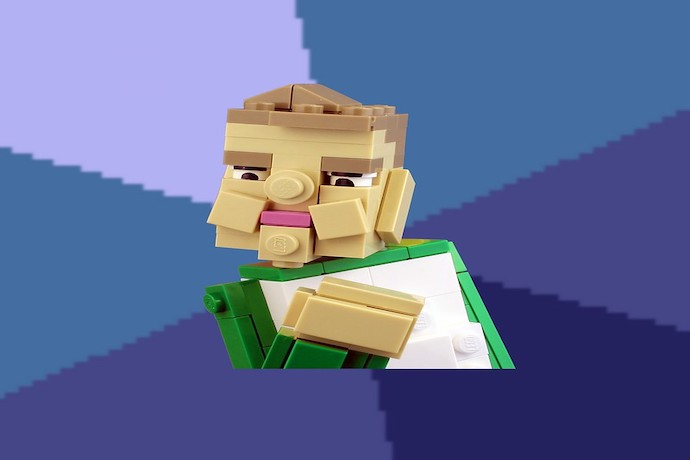Explore the scientific origins of memes!Interested but not ready to subscribe? Sign-up for our free monthly email newsletter with curated site content and a new issue email announcement that we send every two months.

No, thanks!